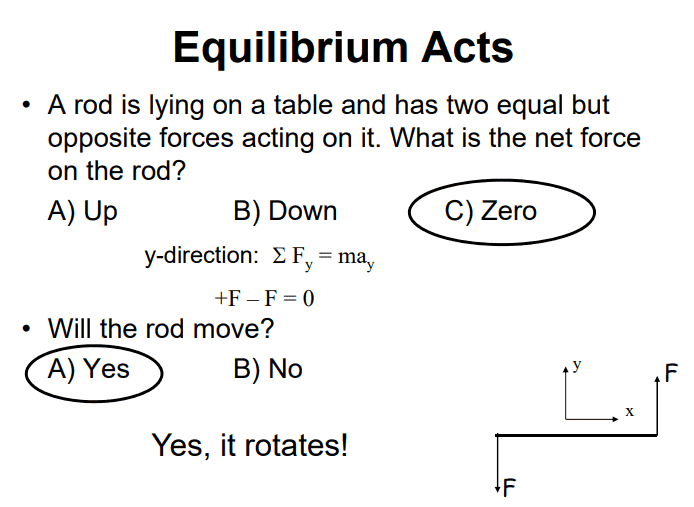# Will it rotate?

SakuRERE

## Homework Statement## Homework Equations

torque= F*r*sintheta
total force on y= 0

## The Attempt at a Solution

how come it will rotate in this situation?? espicially that he is ignoring the weight force of the rod! i knew that i ignored the mass of the rod when he said total force on y= F -F. if there is a mass it should be total force= F-f-mg
and so it will rotate!

#### Attachments

Homework Helper
how come it will rotate in this situation?? espicially that he is ignoring the weight force of the rod! i knew that i ignored the mass of the rod when he said total force on y= F -F. if there is a mass it should be total force= F-f-mg
and so it will rotate!
The rod is lying on a table. If you want to account for gravity, there is another force that you also need to account for...

•SakuRERE
Staff Emeritus
Homework Helper
Gold Member
espicially that he is ignoring the weight force of the rod!
The rod is flat on the table, the weight is acting perpendicular to the table and is offset by the normal force from the table. The rod in your image is seen from above, both forces and the rod are to be considered to be in the horizontal plane. The vertical direction is therefore irrelevant.

•SakuRERE and jbriggs444
SakuRERE
The rod is lying on a table. If you want to account for gravity, there is another force that you also need to account for...
The rod is flat on the table, the weight is acting perpendicular to the table and is offset by the normal force from the table. The rod in your image is seen from above, both forces and the rod are to be considered to be in the horizontal plane. The vertical direction is therefore irrelevant.
ohh yeaah, thanks everyone. i didn't focus on that point that is layning in the table!
so okay, i got the idea of the total f is equal to zero by the two forces, but still, why we have a torque? why there is rotation when the two forces are with the same magnitude and opposite to each other. we must have total torque=0 or what?

SakuRERE
ohh yeaah, thanks everyone. i didn't focus on that point that is layning in the table!
so okay, i got the idea of the total f is equal to zero by the two forces, but still, why we have a torque? why there is rotation when the two forces are with the same magnitude and opposite to each other. we must have total torque=0 or what?
and regarding the pivot point, i think it's the one who will decide if it will rotate or not, i mean if it was at the center of the rod than there should be not rotation, but if the pivot is not in the center (not mentioned) then the distance of each force to the pivot won't be same so then i would say there rotation. but the one answering the question didn't specify any thing !

Staff Emeritus
Homework Helper
Gold Member
The force sum is zero, but the torque is not. This is due to the forces not having the same line of application. If you compute the torque of each force relative to the centre of the rod, you will find that both of the contributions act in the same direction.

•SakuRERE
Staff Emeritus
•